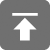# 工学1号馆

home

## Project Euler 73–Counting fractions in a range

Wu Yudong    August 20, 2018     欧拉计划   645

Counting fractions in a range

Consider the fraction, n/d, where n and d are positive integers. If n < d and HCF(n,d)=1, it is called a reduced proper fraction.

If we list the set of reduced proper fractions for d ≤ 8 in ascending order of size, we get:

1/8, 1/7, 1/6, 1/5, 1/4, 2/7, 1/3, 3/8, 2/5, 3/7, 1/2, 4/7, 3/5, 5/8, 2/3, 5/7, 3/4, 4/5, 5/6, 6/7, 7/8
It can be seen that there are 3 fractions between 1/3 and 1/2.

How many fractions lie between 1/3 and 1/2 in the sorted set of reduced proper fractions for d ≤ 12,000?

1/8, 1/7, 1/6, 1/5, 1/4, 2/7, 1/3, 3/8, 2/5, 3/7, 1/2, 4/7, 3/5, 5/8, 2/3, 5/7, 3/4, 4/5, 5/6, 6/7, 7/8

//（Problem 73）Counting fractions in a range
// Completed on Wed, 19 Feb 2014, 16:34
// Language: C11
//
// 版权所有（C）wuyudong
// 博客地址：http://www.wuyudong.com

#include<stdio.h>
#define N 12000

int gcd(int a, int b)  //求最大公约数函数
{
int r;
while(b) {
r = a % b;
a = b;
b = r;
}
return a;
}

void solve()
{
int a, b, i, j, ans;
ans = 0;
for(i = 5; i <= N; i++) {
a = i / 3;  b = i / 2;
for(j = a + 1; j < b + 1; j++) {
if(gcd(i, j) == 1)
ans++;
}
}
printf("%d\n", ans);
}

int main()
{
solve();
return 0;
}